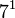# Groups of order 1400

## Contents

See pages on algebraic structures of order 1400| See pages on groups of a particular order

## Statistics at a glance

The order 1400 has prime factorization:$1400 = 2^3 \cdot 5^2 \cdot 7^1 = 8 \cdot 25 \cdot 7$

Quantity Value Explanation
Total number of groups up to isomorphism 153
Number of abelian groups up to isomorphism 6 (number of abelian groups of order$2^3$)$\times$ (number of abelian groups of order$5^2$)$\times$ (number of abelian groups of order$7^1$) = (number of unordered integer partitions of 3)$\times$ (number of unordered integer partitions of 2)$\times$ (number of unordered integer partitions of 1) =$3 \times 2 \times 1 = 6$. See classification of finite abelian groups and structure theorem for finitely generated abelian groups.
Number of nilpotent groups up to isomorphism 10 (number of groups of order 8)$\times$ (number of groups of order 25)$\times$ (number of groups of order 7) =$5 \times 2 \times 1 = 10$. See number of nilpotent groups equals product of number of groups of order each maximal prime power divisor, which in turn follows from equivalence of definitions of finite nilpotent group.
Number of solvable groups up to isomorphism 153 all groups of this order are solvable
Number of simple non-abelian groups up to isomorphism 0

## GAP implementation

The order 1400 is part of GAP's SmallGroup library. Hence, any group of order 1400 can be constructed using the SmallGroup function by specifying its group ID. Also, IdGroup is available, so the group ID of any group of this order can be queried.

Further, the collection of all groups of order 1400 can be accessed as a list using GAP's AllSmallGroups function.

Here is GAP's summary information about how it stores groups of this order, accessed using GAP's SmallGroupsInformation function:

gap> SmallGroupsInformation(1400);

There are 153 groups of order 1400.
They are sorted by their Frattini factors.
1 has Frattini factor [ 70, 1 ].
2 has Frattini factor [ 70, 2 ].
3 has Frattini factor [ 70, 3 ].
4 has Frattini factor [ 70, 4 ].
5 has Frattini factor [ 140, 5 ].
6 has Frattini factor [ 140, 6 ].
7 - 13 have Frattini factor [ 140, 7 ].
14 - 18 have Frattini factor [ 140, 8 ].
19 - 23 have Frattini factor [ 140, 9 ].
24 - 28 have Frattini factor [ 140, 10 ].
29 - 31 have Frattini factor [ 140, 11 ].
32 has Frattini factor [ 280, 32 ].
33 has Frattini factor [ 280, 33 ].
34 has Frattini factor [ 280, 34 ].
35 has Frattini factor [ 280, 35 ].
36 has Frattini factor [ 280, 36 ].
37 has Frattini factor [ 280, 37 ].
38 has Frattini factor [ 280, 38 ].
39 has Frattini factor [ 280, 39 ].
40 has Frattini factor [ 280, 40 ].
41 has Frattini factor [ 350, 5 ].
42 has Frattini factor [ 350, 6 ].
43 has Frattini factor [ 350, 7 ].
44 has Frattini factor [ 350, 8 ].
45 has Frattini factor [ 350, 9 ].
46 has Frattini factor [ 350, 10 ].
47 has Frattini factor [ 700, 18 ].
48 has Frattini factor [ 700, 19 ].
49 has Frattini factor [ 700, 20 ].
50 has Frattini factor [ 700, 21 ].
51 has Frattini factor [ 700, 22 ].
52 has Frattini factor [ 700, 23 ].
53 has Frattini factor [ 700, 24 ].
54 has Frattini factor [ 700, 25 ].
55 - 61 have Frattini factor [ 700, 26 ].
62 - 68 have Frattini factor [ 700, 27 ].
69 - 73 have Frattini factor [ 700, 28 ].
74 - 80 have Frattini factor [ 700, 29 ].
81 - 85 have Frattini factor [ 700, 30 ].
86 - 90 have Frattini factor [ 700, 31 ].
91 - 95 have Frattini factor [ 700, 32 ].
96 - 100 have Frattini factor [ 700, 33 ].
101 - 105 have Frattini factor [ 700, 34 ].
106 - 110 have Frattini factor [ 700, 35 ].
111 - 113 have Frattini factor [ 700, 36 ].
114 - 153 have trivial Frattini subgroup.

For the selection functions the values of the following attributes
are precomputed and stored:
IsAbelian, IsNilpotentGroup, IsSupersolvableGroup, IsSolvableGroup,
LGLength, FrattinifactorSize and FrattinifactorId.

This size belongs to layer 5 of the SmallGroups library.
IdSmallGroup is available for this size.